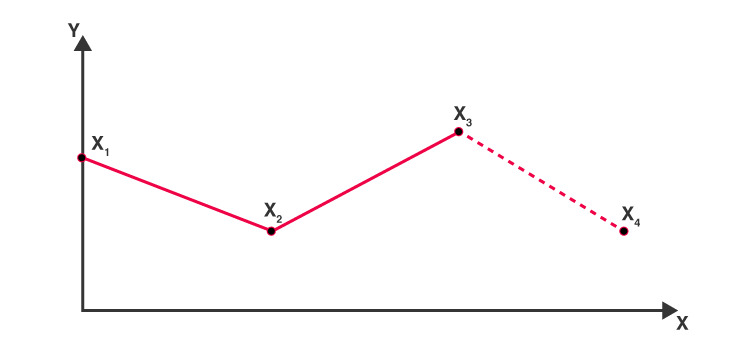Checkout JEE MAINS 2022 Question Paper Analysis : Checkout JEE MAINS 2022 Question Paper Analysis :

# Extrapolation

In Statistics, Extrapolation is a process of estimating the value beyond the distinct range of the given variable based on its relationship with another variable. It is an important concept not only in Mathematics but also in other disciplines like Psychology, Sociology, Statistics, etc., with some categorical data. Here, we will discuss in detail about definition, formula and examples of extrapolation. One more important concept is an interpolation, which is defined as an estimation between the given observations or data.

## Extrapolation Definition

Extrapolation is defined as an estimation of a value based on extending the known series or factors beyond the area that is certainly known. In other words, extrapolation is a method in which the data values are considered as points such as x1, x2, ….., xn. It commonly exists in statistical data very often, if that data is sampled periodically and it approximates the next data point. One such example is when you are driving, you usually extrapolate about road conditions beyond your sight.

## What is Extrapolation Statistics?

Extrapolation is a statistical method beamed at understanding the unknown data from the known data. It tries to predict future data based on historical data. For example, estimating the size of a population after a few years based on the current population size and its rate of growth.

## Extrapolation Methods

Extrapolation is classified into three types, namely

• Linear extrapolation
• Conic extrapolation
• Polynomial Extrapolation

Let us discuss the three types of extrapolation in detail.

### Linear Extrapolation

For any linear function, linear extrapolation provides a good result when the point to be predicted is not too far from the given data. It is usually done by drawing the tangent line at the endpoint of the given graph and that will be extended beyond the limit.

### Polynomial Extrapolation

A polynomial curve can be created with the help of entire known data or near the endpoints. This method is typically done using Lagrange interpolation or Newton’s system of finite series that provides the data. The final polynomial is used to extrapolate the data using the associated endpoints.

### Conic Extrapolation

A conic section can be created with the help of five points nearer to the end of the given (i.e. known) data. The conic section will curve back on itself if it is a circle or ellipse. But for parabola or hyperbola, the curve will not back on itself because it is relative to the X-axis.

## Extrapolation Formula

Let us consider the two endpoints in a linear graph (x1, y1) and (x2, y2) where the value of the point “x” is to be extrapolated, and then the extrapolation formula is given as

$$\begin{array}{l}y(x)= y_{1}+\frac{x-x_{1}}{x_{2}-x_{1}}(y_{2}-y_{1})\end{array}$$

## Extrapolation Graph

As we know, extrapolation is a process of predicting the data point about the outside of a curve when a few points are given. In the example given below, the known data are x1, x2, x3. Finding the point x4 is known as extrapolation point.### Extrapolation Example

Question:

The two given points that lie on the straight line is (1, 5) and (4, 10). Determine the value of y at x = 5 on the straight line using a linear extrapolation method.

Solution:

Given: x1 = 1 ,y1 = 5

and x2 = 4, y2 = 10

The linear extrapolation formula is given as;

$$\begin{array}{l}y(x)= y_{1}+\frac{x-x_{1}}{x_{2}-x_{1}}(y_{2}-y_{1})\end{array}$$

Substitute the known values in the given formula,

y(5) = 5 +[(5-1)/(4-1)](10-5)

y(5) = 5 + (4/3) (5)

y(5) = 5 + 6.67

y(5)= 11.67

Therefore y(5) = 11.67

For more maths-related concepts, stay tuned with BYJU’S – The Learning App and also watch personalised videos to learn with ease.